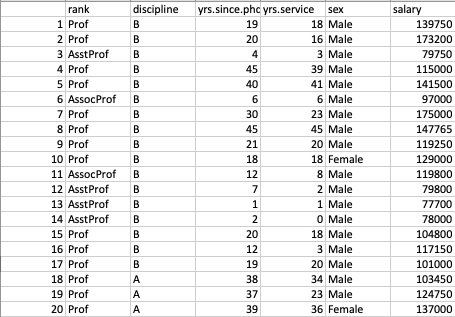# Find Mean Absolute Deviation Of A Pandas.series

## Mean Absolute Deviation:

• In Statistics parlance, deviation means how far a value in the distribution is away from the mean.
• The deviation from the mean for a specific value present in the distribution could be either positive or negative. If the sign is removed from the deviation and only the magnitude is considered it is called as Absolute Deviation.
• The average value of all the Absolute Deviations of a distribution is called as the Mean Absolute Deviation.
• Since the Absolute Deviations from which the Mean Absolute Deviation is computed are all without a sign, the Mean Absolute Deviation also does not have a sign.
• The Mean Absolute Deviation tells how far, on an average the values in the distribution are away from the average or mean. It is a measure of dispersion.
• Some view that Mean Absolute Deviation provides a clear understanding of the dispersion of values than the Standard Deviation.

## Finding Mean Absolute Deviation (MAD) for a pandas.Series in Python:

• The pandas.Series class in Python implements a method, Series.mad() that  calculates the Mean Absolute Deviation for the distribution present in a pandas Series.
• The Python example loads the salary details of Professors and Assistant Professors into a DataFrame(Data Courtesy:R Datasets).## Example:

 # Example Python program that calculates the Mean Absolute Deviation(MAD) of a # distribution present in a pandas.Series (Data Courtesy: R Datasets) import pandas as pds   path        = "./Salaries.csv" dataFrame   = pds.read_csv(path); asstProf    = dataFrame.loc[dataFrame['rank'] == "AsstProf"];   # Get the salaries of Assistant Professors salaries    = asstProf["salary"];   # Get the Mean Absolute Deviation of salaries on Assistant Professors grade mad_ap = salaries.mad(); print("Mean Absolute Deviation of Assistant Professor Salaries:"); print(round(mad_ap,2));

## Output:

 Mean Absolute Deviation of Assistant Professor Salaries: 6982.01